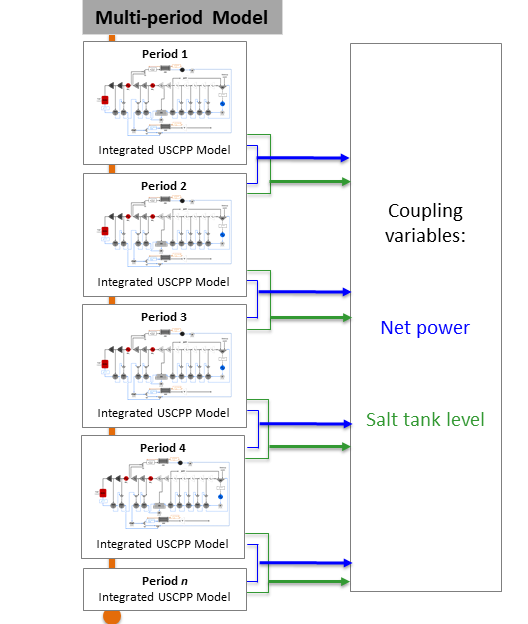# Multiperiod Integrated Ultra-Supercritical Power Plant

The Multiperiod Integrated Ultra-Supercritical Power Plant is an example model to determine the optimal schedule and operation of a pulverized coal-fired ultra-supercritical power plant integrated with thermal energy storage system (TES) for given electricity prices over a time horizon. The multiperiod model uses the Integrated Ultra-Supercritical Power Plant model for each period in the horizon. A more detailed description of the model is given in the sections below.

## Model Structure

The multiperiod power plant model is developed by creating multiple instances of Integrated Ultra-Supercritical Power Plant model, where every instance is indexed by a time period, along with two coupling variables to link each time period. These coupling variables are: the optimal power produced by the power plant and the amount of storage material available at the end of each time period. A scheme showing how the multiperiod model is constructed is shown in the figure below:## Degrees of Freedom

The multiperiod model has a total of 4 $$n$$ degrees of freedom, where $$n$$ represents the number of time periods. The degrees of freedom for each time period are listed below:

1. Boiler feed water flow ($$boiler.inlet.flow_-mol$$),

2. Steam flow to charge heat exchanger ($$HXC.inlet_-1.flow_-mol$$)

3. Condensate flow to discharge heat exchanger ($$HXD.inlet_-2.flow_-mol$$),

4. Cooler enthalpy at outlet ($$cooler.outlet.enth_-mol$$)

## Notable Variables

Variable Name

Description

$$NetPower_t$$

Net power out from the power plant at each time period in MW

$$SaltInventory_t$$

Solar salt inventory at each time period in kg

## Notable Constraints

1. Power plant ramping is limited by including the following equations for a given $$ramp_-rate$$:

$NetPower_{t-1} - ramp_-rate \leq NetPower_t$
$NetPower_{t-1} + ramp_-rate \geq NetPower_t$
1. The salt inventory $$SaltInventory$$ is given by the hot salt and total salt material balances, the latter involving a fixed amount of salt $$total_-salt$$. The material balances are shown in the following equations:

$HotSaltInventory_t = HotSaltInventory_{t-1} + F^{charge}_{salt, outlet} - F^{discharge}_{salt, inlet}$
$total_-salt = HotSaltInventory_t + ColdSaltInventory_t$

Note that the notable constraints and variables in the multiperiod model also consider the notable variables and constraints given in Integrated Ultra-Supercritical Power Plant.